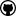org.dllearner.algorithms.ocel

## Class MultiHeuristic

• All Implemented Interfaces:
Comparator<ExampleBasedNode>, ExampleBasedHeuristic, Component, Heuristic<ExampleBasedNode>

```public class MultiHeuristic
extends Object
implements ExampleBasedHeuristic, Component```
This heuristic combines the following criteria to assign a double score value to a node:
• quality/accuracy of a concept (based on the full training set, not the negative example coverage as the flexible heuristic)
• horizontal expansion
• accuracy gain: The heuristic takes into account the accuracy difference between a node and its parent. If there is no gain (even though we know that the refinement is proper) it is unlikely (although not excluded) that the refinement is a necessary path to take towards a solution.
The heuristic has two parameters:
• expansion penalty factor: describes how much accuracy gain is worth an increase of horizontal expansion by one (typical value: 0.01)
• gain bonus factor: describes how accuracy gain should be weighted versus accuracy itself (typical value: 1.00)
The value of a node is calculated as follows:

```value = accuracy + gain bonus factor * accuracy gain - expansion penalty factor * horizontal expansion - node children penalty factor * number of children of node```

`accuracy = (TP + TN)/(P + N)`

``` TP = number of true positives (= covered positives) TN = number of true negatives (= nr of negatives examples - covered negatives) P = number of positive examples N = number of negative examples ```

Author:
Jens Lehmann
• ### Constructor Summary

Constructors
Constructor and Description
`MultiHeuristic()`
```MultiHeuristic(int nrOfPositiveExamples, int nrOfNegativeExamples)```
Deprecated.
```MultiHeuristic(int nrOfPositiveExamples, int nrOfNegativeExamples, double negativeWeight, double startNodeBonus, double expansionPenaltyFactor, int negationPenalty)```
• ### Method Summary

All Methods
Modifier and Type Method and Description
`int` ```compare(ExampleBasedNode node1, ExampleBasedNode node2)```
`double` `getExpansionPenaltyFactor()`
`double` `getGainBonusFactor()`
`int` `getNegationPenalty()`
`double` `getNegativeWeight()`
`double` `getNodeChildPenalty()`
`double` `getNodeScore(ExampleBasedNode node)`
`static double` ```getNodeScore(ExampleBasedNode node, int nrOfPositiveExamples, int nrOfNegativeExamples, double negativeWeight, double startNodeBonus, double expansionPenaltyFactor, int negationPenalty)```
`int` `getNrOfExamples()`
`int` `getNrOfNegativeExamples()`
`double` `getStartNodeBonus()`
`void` `init()`
Method to be called after the component has been configured.
`void` `setExpansionPenaltyFactor(double expansionPenaltyFactor)`
`void` `setGainBonusFactor(double gainBonusFactor)`
`void` `setNegationPenalty(int negationPenalty)`
`void` `setNegativeWeight(double negativeWeight)`
`void` `setNodeChildPenalty(double nodeChildPenalty)`
`void` `setNrOfExamples(int nrOfExamples)`
`void` `setNrOfNegativeExamples(int nrOfNegativeExamples)`
`void` `setStartNodeBonus(double startNodeBonus)`
• ### Methods inherited from class java.lang.Object

`equals, getClass, hashCode, notify, notifyAll, toString, wait, wait, wait`
• ### Methods inherited from interface java.util.Comparator

`comparing, comparing, comparingDouble, comparingInt, comparingLong, equals, naturalOrder, nullsFirst, nullsLast, reversed, reverseOrder, thenComparing, thenComparing, thenComparing, thenComparingDouble, thenComparingInt, thenComparingLong`
• ### Constructor Detail

• #### MultiHeuristic

```@Deprecated
public MultiHeuristic(int nrOfPositiveExamples,
int nrOfNegativeExamples)```
Deprecated.
• #### MultiHeuristic

```public MultiHeuristic(int nrOfPositiveExamples,
int nrOfNegativeExamples,
double negativeWeight,
double startNodeBonus,
double expansionPenaltyFactor,
int negationPenalty)```
• #### MultiHeuristic

`public MultiHeuristic()`
• ### Method Detail

• #### init

```public void init()
throws ComponentInitException```
Description copied from interface: `Component`
Method to be called after the component has been configured. Implementation of components can overwrite this method to perform setup and initialisation tasks for this component.
Specified by:
`init` in interface `Component`
Throws:
`ComponentInitException` - This exception is thrown if any exceptions occur within the initialisation process of this component. As component developer, you are encouraged to re-throw occurring exception as ComponentInitException and giving an error message as well as the actually exception by using the constructor `ComponentInitException(String, Throwable)`.
• #### compare

```public int compare(ExampleBasedNode node1,
ExampleBasedNode node2)```
Specified by:
`compare` in interface `Comparator<ExampleBasedNode>`
• #### getNodeScore

`public double getNodeScore(ExampleBasedNode node)`
Specified by:
`getNodeScore` in interface `Heuristic<ExampleBasedNode>`
• #### getNodeScore

```public static double getNodeScore(ExampleBasedNode node,
int nrOfPositiveExamples,
int nrOfNegativeExamples,
double negativeWeight,
double startNodeBonus,
double expansionPenaltyFactor,
int negationPenalty)```
• #### getExpansionPenaltyFactor

`public double getExpansionPenaltyFactor()`
• #### setExpansionPenaltyFactor

`public void setExpansionPenaltyFactor(double expansionPenaltyFactor)`
• #### getNrOfNegativeExamples

`public int getNrOfNegativeExamples()`
• #### setNrOfNegativeExamples

`public void setNrOfNegativeExamples(int nrOfNegativeExamples)`
• #### getNrOfExamples

`public int getNrOfExamples()`
• #### setNrOfExamples

`public void setNrOfExamples(int nrOfExamples)`
• #### getGainBonusFactor

`public double getGainBonusFactor()`
• #### setGainBonusFactor

`public void setGainBonusFactor(double gainBonusFactor)`
• #### getNodeChildPenalty

`public double getNodeChildPenalty()`
• #### setNodeChildPenalty

`public void setNodeChildPenalty(double nodeChildPenalty)`
• #### getStartNodeBonus

`public double getStartNodeBonus()`
• #### setStartNodeBonus

`public void setStartNodeBonus(double startNodeBonus)`
• #### getNegativeWeight

`public double getNegativeWeight()`
• #### setNegativeWeight

`public void setNegativeWeight(double negativeWeight)`
• #### getNegationPenalty

`public int getNegationPenalty()`
• #### setNegationPenalty

`public void setNegationPenalty(int negationPenalty)`DL-Learner is licenced under the terms of the GNU General Public License.